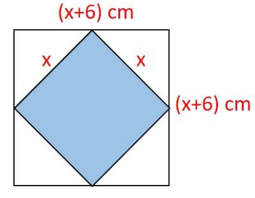Maths-
General
Easy

Question

# What is the total area of four white triangles if 𝑥 = 12 𝑐𝑚?Hint:

## The correct answer is: 180 cm2.

### The area of the outer square of side x+6 cm = (x+6)2(x+6)2  = (x+6)(x+6) = x(x+6) +6(x+6)= x(x) + x(6) +6(x) +6(6)= x2 + 6x + 6x + 36= x2 + 12x + 36The area of the inner square of side x cm = x2Now, Total area of four white triangles = Area of the outer square - area of the inner square= x2 + 12x + 36 - x2= 12x + 36Given that x =12 cmSo, Total area of four white triangles = 12(12) + 36= 144 + 36 = 180 cm2Final Answer: Hence, the total area of four white triangles is 180 cm2.#### With Turito Foundation.#### Get an Expert Advice From Turito.